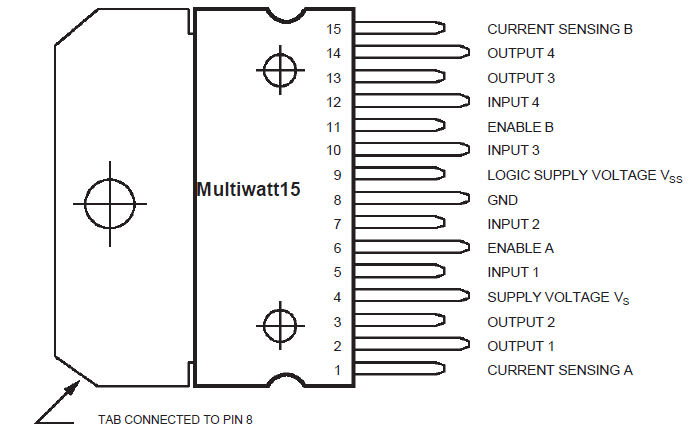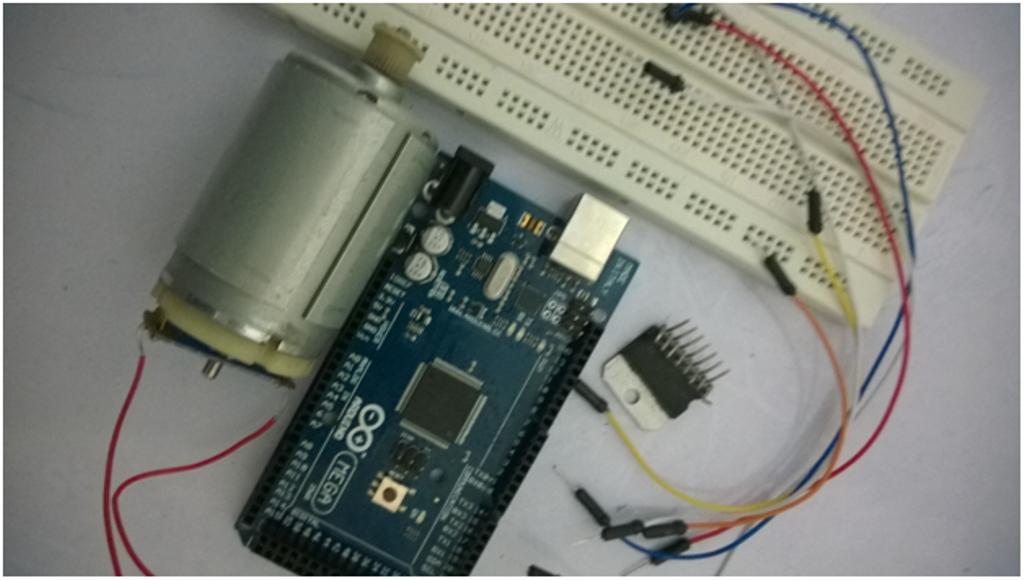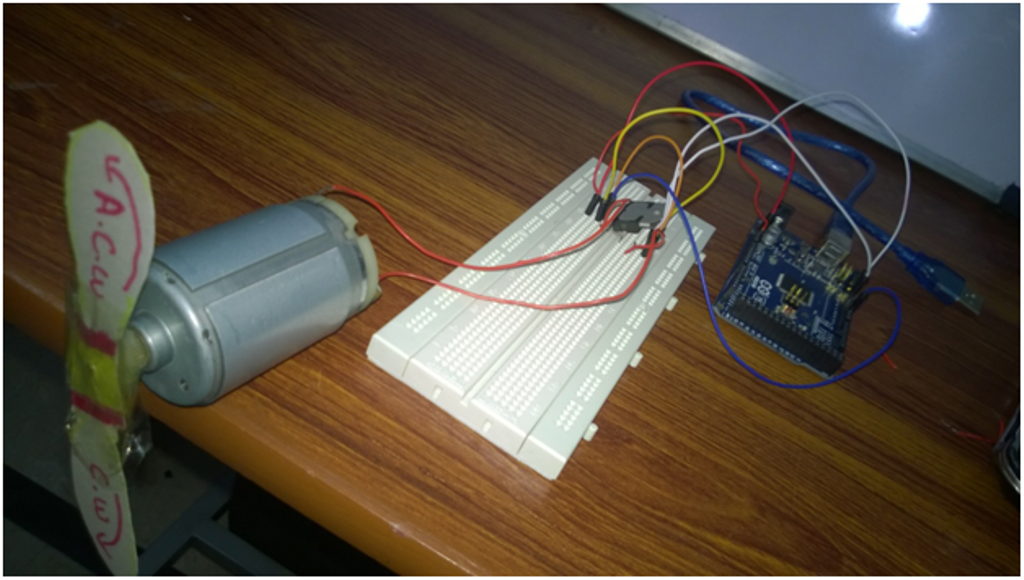Project

# How To Control a DC Motor with an Arduino

July 07, 2018 by Tim Youngblood

## By connecting an L298 bridge IC to an Arduino, you can control a DC motor.

By connecting an L298 bridge IC to an Arduino, you can control a DC motor.

A direct current, or DC, motor is the most common type of motor. DC motors normally have just two leads, one positive and one negative. If you connect these two leads directly to a battery, the motor will rotate. If you switch the leads, the motor will rotate in the opposite direction.

To control the direction of the spin of DC motor, without changing the way that the leads are connected, you can use a circuit called an H-Bridge. An H bridge is an electronic circuit that can drive the motor in both directions. H-bridges are used in many different applications, one of the most common being to control motors in robots. It is called an H-bridge because it uses four transistors connected in such a way that the schematic diagram looks like an "H."

You can use discrete transistors to make this circuit, but for this tutorial, we will be using the L298 H-Bridge IC. The L298 can control the speed and direction of DC motors and stepper motors and can control two motors simultaneously. Its current rating is 2A for each motor. At these currents, however, you will need to use heat sinks.

The pinouts for the L298 are shown below. You can find a datasheet the L298 at http://www.tech.dmu.ac.uk/~mgongora/Resources/L298N.pdf.

#### L298 Pinout (top view)#### Hardware Required

• 1 x L298 bridge IC
• 1 x DC motor
• 1 x Arduino Mega2560
• 10 x jumper wiresThe schematic above shows how to connect the L298 IC to control two motors. There are three input pins for each motor, including Input1 (IN1), Input2 (IN2), and Enable1 (EN1) for Motor1 and Input3, Input4, and Enable2 for Motor2.

Since we will be controlling only one motor in this tutorial, we will connect the Arduino to IN1 (pin 5), IN2 (pin 7), and Enable1 (pin 6) of the L298 IC. Pins 5 and 7 are digital, i.e. ON or OFF inputs, while pin 6 needs a pulse-width modulated (PWM) signal to control the motor speed.

The following table shows which direction the motor will turn based on the digital values of IN1 and IN2.

IN1 IN2 MOTOR
0 0 BRAKE
1 0 FORWARD
0 1 BACKWARD
1 1 BRAKE

IN1 pin of the L298 IC is connected to pin 8 of the Arduino while IN2 is connected to pin 9. These two digital pins of Arduino  control the direction of the motor. The EN A pin of IC is connected to the PWM pin 2 of Arduino. This will control the speed of the motor.

To set the values of Arduino pins 8 and 9, we will use the digitalWrite() function, and to set the value of pin 2, we will use the using analogWrite() function.

Below is a photo of the set up.#### ​Code

const int pwm = 2 ;	//initializing pin 2 as pwm
const int in_1 = 8 ;
const int in_2 = 9 ;

//For providing logic to L298 IC to choose the direction of the DC motor

void setup()
{
pinMode(pwm,OUTPUT) ;  	//we have to set PWM pin as output
pinMode(in_1,OUTPUT) ; 	//Logic pins are also set as output
pinMode(in_2,OUTPUT) ;
}

void loop()
{
//For Clock wise motion , in_1 = High , in_2 = Low

digitalWrite(in_1,HIGH) ;
digitalWrite(in_2,LOW) ;
analogWrite(pwm,255) ;

/*setting pwm of the motor to 255
we can change the speed of rotaion
by chaning pwm input but we are only
using arduino so we are using higest
value to driver the motor  */

//Clockwise for 3 secs
delay(3000) ;

//For brake
digitalWrite(in_1,HIGH) ;
digitalWrite(in_2,HIGH) ;
delay(1000) ;

//For Anti Clock-wise motion - IN_1 = LOW , IN_2 = HIGH
digitalWrite(in_1,LOW) ;
digitalWrite(in_2,HIGH) ;
delay(3000) ;

//For brake
digitalWrite(in_1,HIGH) ;
digitalWrite(in_2,HIGH) ;
delay(1000) ;
}



dc_motor.ino.zip

#### Arduino Motor Control Setup

1. Connect 5V and ground of the IC to 5V and ground of Arduino.
2. Connect the motor to pins 2 and 3 of the IC.
3. Connect IN1 of the IC to pin 8 of Arduino.
4. Connect IN2 of the IC to pin 9 of Arduino.
5. Connect EN1 of IC to pin 2 of Arduino.
6. Connect SENS A pin of IC to the ground.
7. Connect the Arduino using Arduino USB cable and upload the program to the Arduino using Arduino IDE software or Arduino Web Editor.
8. Provide power to the Arduino board using power supply, battery or USB cable.

The motor should now run first in the clockwise (CW) direction for 3 seconds and then counter-clockwise (CCW) for 3 seconds.

#### Video

Give this project a try for yourself! Get the BOM.•Clint Zang June 27, 2015

OK. Understandable, however I want to beef it up to control a treadmill motor. Anybody done that with success? I’d like to know how.

Like.
• M
mertcapanoglu August 27, 2015

This article doesn’t say anything about the Vs(supply voltage) of the IC, just the logic supply. I’m guessing using a high power dc motor would fry the usb and possibly the motherboard of the computer.

Like.
• E
EdFone October 31, 2015